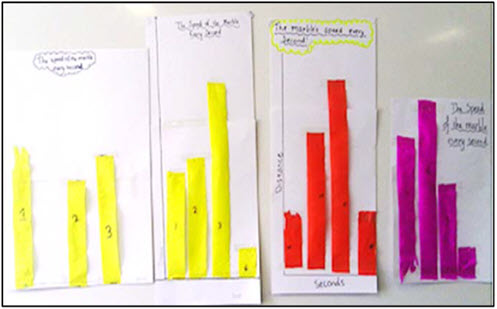Go to website

# reSolve: Modelling Motion: Years 5 and 6

In this sequence of lessons students use a streamer graph to track speed and develop the concept of speed as time per unit travelled. They experiment with rolling a ball up and down a slope and gather data to make predictions. Each lesson is outlined in detail including curriculum links, vocabulary, materials needed, sample answers, discussion points and student resources. This sequence is part of the reSolve: Mathematics by Inquiry program.

Year level(s) Year 5, Year 6
Audience Teacher
Purpose Teaching resource
Teaching strategies and pedagogical approaches Mathematics investigation
Keywords speed, data, time, graph

## Curriculum alignment

Curriculum connections Numeracy, Science, Technologies, Critical and creative thinking
Strand and focus Measurement, Build understanding, Apply understanding
Topics Using units of measurement, Data representation and interpretation
AC: Mathematics (V9.0) content descriptions
AC9M5ST01
Acquire, validate and represent data for nominal and ordinal categorical and discrete numerical variables to address a question of interest or purpose using software including spreadsheets; discuss and report on data distributions in terms of highest frequency (mode) and shape, in the context of the data

AC9M6ST01
Interpret and compare data sets for ordinal and nominal categorical, discrete and continuous numerical variables using comparative displays or visualisations and digital tools; compare distributions in terms of mode, range and shape

AC9M5M01
Choose appropriate metric units when measuring the length, mass and capacity of objects; use smaller units or a combination of units to obtain a more accurate measure

AC9M5SP03
Describe and perform translations, reflections and rotations of shapes, using dynamic geometric software where appropriate; recognise what changes and what remains the same, and identify any symmetries

AC9M5N07
Solve problems involving division, choosing efficient strategies and using digital tools where appropriate; interpret any remainder according to the context and express results as a whole number, decimal or fraction

AC9M5ST03
Plan and conduct statistical investigations by posing questions or identifying a problem and collecting relevant data; choose appropriate displays and interpret the data; communicate findings within the context of the investigation

AC9M5ST01
Acquire, validate and represent data for nominal and ordinal categorical and discrete numerical variables to address a question of interest or purpose using software including spreadsheets; discuss and report on data distributions in terms of highest frequency (mode) and shape, in the context of the data

AC9M6M01
Convert between common metric units of length, mass and capacity; choose and use decimal representations of metric measurements relevant to the context of a problem

AC9M6N09
Use mathematical modelling to solve practical problems, involving rational numbers and percentages, including in financial contexts; formulate the problems, choosing operations and efficient calculation strategies, and using digital tools where appropriate; interpret and communicate solutions in terms of the situation, justifying the choices made

AC9M5N04
Recognise that 100% represents the complete whole and use percentages to describe, represent and compare relative size; connect familiar percentages to their decimal and fraction equivalents

Interpreting fractions (P6, P8)
Interpreting and representing data (P4)
Multiplicative strategies (P7, P8)
Number and place value (P8)
Proportional thinking (P1)
Understanding geometric properties (P5)
Understanding money (P8)
Understanding units of measurement (P8)